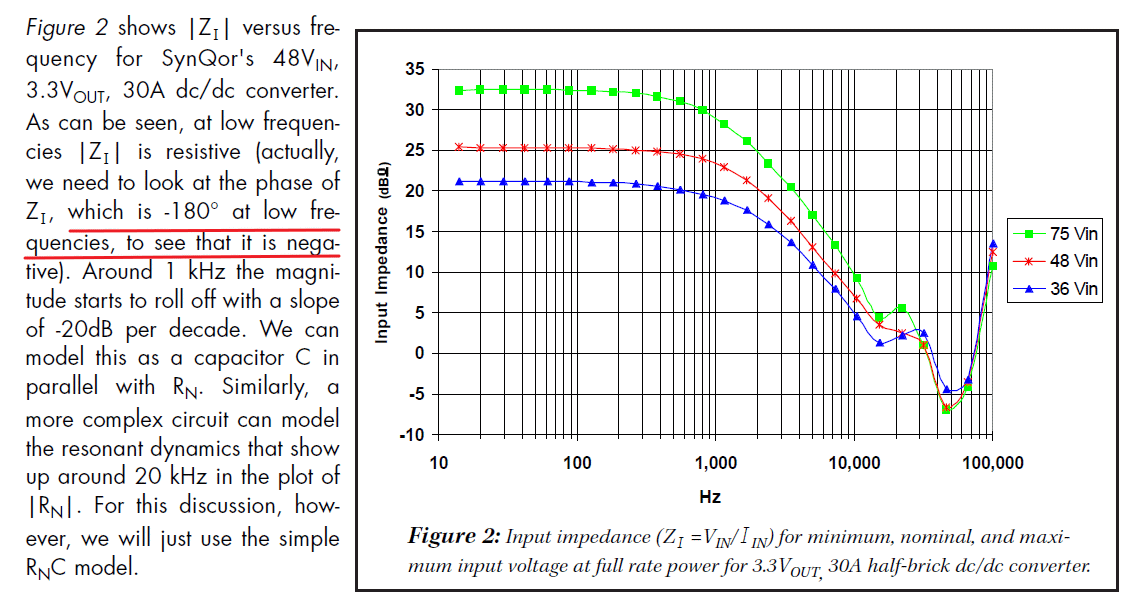# Ensuring Stable DC Power Delivery To Switching Loads

Quote of the Day

Life is never made unbearable by circumstances, but only by lack of meaning and purpose.

## Introduction

I presented a seminar over lunch today on short-range DC power distribution, which I believe is one of the most exciting areas in electronics today.  AC power distribution has dominated power engineering since the "War of the Currents" ended with Westinghouse's AC system winning a decisive victory over Edison's DC system back in the 1890s. Starting in 1930s Europe, high-voltage DC distribution has slowly gained a foothold in some long-haul, high power distribution applications, but most power distribution has continued to be dominated by AC.

We are now seeing a resurgence in low-voltage DC power for use in short-range power distribution because of recent technology changes:

• Increasing use of photovoltaics, which produce DC.
• Increasing use of LED lighting, which can be powered more efficiently by DC.
• Desire to use network cable to distribute both power and data (e.g. PoE).
• Desire to reduce installation costs by eliminating the expense associated with ensuring that AC wiring is safe (e.g. conduit, heavy gauge wire, labor using highly-trained electricians, etc).

One issue that needs to be addressed is how to ensure the stability of a DC distribution when it is driving loads composed switching power supplies, which have a rather complicated input impedance function. One common approach is to apply the Middlebrook stability criterion, which provides a sufficient condition for a stable DC network. In this blog post, I will be discussing the derivation and application of the Middlebrook stability criterion.

My raw Mathcad file is included here (with PDF) if you wish to work through the examples yourself.

## Background

### History

RD Middlebrook published his criterion in the journal article "Input Filter Considerations, in Design and Application of Switching Regulators",  IEEE Industry Applications Society Annual Meeting, October, 1976. The Middlebrook criterion is commonly used because it is simple to apply, however it has relatively high implementation cost. For a discussion of the alternatives, see this presentation.

The Middlebrook criterion is also known as an impedance ratio criterion. I will demonstrate why it is called an impedance ratio criterion in Figure 5.

### Middlebrook Criterion Statement

The following discussion summarizes a longer paper that I include here.

Figure 3 shows how power engineers usually define the source and input impedances of a power system.

Using the impedance definitions illustrated in Figure 3, I usually see the Middlebrook criterion stated as follows:

A system will be locally stable if the magnitude of the input impedance of the load subsystem is larger than the magnitude of the output impedance of source subsystem.

You also often see engineers refer to the criterion's equivalent graphical form:

If the magnitude plots of the source (ZS) and load impedances  (ZL) do not intersect, the system is stable. If the impedance intersection occurs, the system may not be stable. If the impedance magnitude intersection occurs, the system will still be stable providing that the impedance ratio transfer function ZS/ZL satisfies the Nyquist stability test.

Figure 4 shows what a graphical analysis looks like (Source). The yellow cross-hatched region show that there is a potential stability problem with this system. Because the Middlebrook criterion is a sufficient but not necessary condition, more analysis is needed to determine if there is a real problem. In practice, most engineers would not do further analysis but instead would add some form of compensation network to eliminate the yellow cross-hatched region.

One advantage of the graphical approach is that it can be applied to measured impedance data. See Appendix A for an plot of an actual power supply input impedance. The measured data can be quite complex.

## Analysis

### Derivation

Figure 5 shows my pictorial view on how to "prove" the Middlebrook criterion. The process is straightforward:

• Generate a model of the power converter output and the load.

This step defines the critical impedance values (Zo1 and Yi2=1/Zi2) that are used to evaluate the stability of a power distribution system.

• Perform a simple circuit analysis that yields two equations.
• These equations can be represented as a control system graph.

This step defines allows us to apply an existing stability criterion to this specific case.

• This control system graph meets the requirements of the Small Signal Theorem, which provides the stability criterion.

The Small Signal Theorem provides us a sufficient condition for stability, i.e. the system is stable if$\left\| {{{Z}_{{o1}}}\left( {j\cdot \omega } \right)} \right\|\cdot \left\| {{{Y}_{{i2}}}\left( {j\cdot \omega } \right)} \right\|<1$ . This is the key result.

• Express Yi2 as 1/Zi2, which gives us the impedance ratio criterion, i.e.$\frac{{\left\| {{{Z}_{{o1}}}\left( {j\cdot \omega } \right)} \right\|}}{{\left\| {{{Z}_{{i2}}}\left( {j\cdot \omega } \right)} \right\|}}< 1$.

### Worked Example: Uncompensated Case

I found a good paper that illustrated how to apply Middlebrook criterion in practice, and I will work through this paper in detail here.

Figure 6 shows a typical power supply situation and the sufficient conditions for stability in this case (light yellow highlight). This configuration may not be stable, but can often be made stable by adding a lossy capacitor, which I discuss in the following section.

### Worked Example: Lossy Capacitor Compensation

Figure 7 shows how adding a lossy capacitor may stabilize the circuit of Figure 6. The analysis shown in Figure 7 is for a simplified version of Figure 6 – the algebra quickly gets out of control otherwise.

## Conclusion

I have used the Middlebrook stability criterion for years, but have never taken the time to write down a tutorial for my staff. My recent seminar preparation provided me an excuse to finally write down a tutorial.

## Appendix A: Measured Power Supply Input Impedance

Figure 7 shows a graph of an actual power supply input impedance (Source).This entry was posted in Electronics, Power Over Ethernet. Bookmark the permalink.